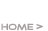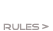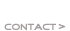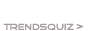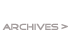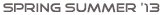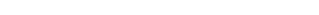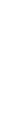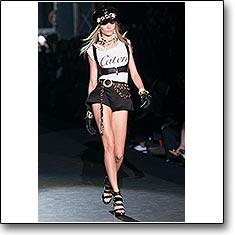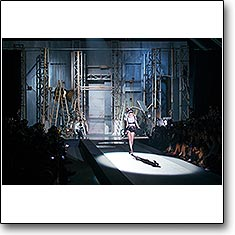D Squared 2 Spring Summer '13 Code: d-squared-s1301 model: Cara Delevingne D Squared 2 Spring Summer '13 Code: d-squared-s1302 D Squared 2 Spring Summer '13 Code: d-squared-s1303 model: Lindsey Wixson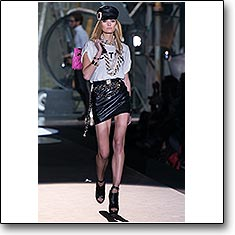D Squared 2 Spring Summer '13 Code: d-squared-s1304 model: Jac Jagaciak D Squared 2 Spring Summer '13 Code: d-squared-s1305 model: Lina Zhang D Squared 2 Spring Summer '13 Code: d-squared-s1306 model: Constance Jablonski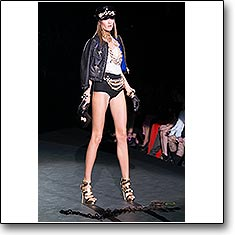D Squared 2 Spring Summer '13 Code: d-squared-s1307 model: Bette Franke D Squared 2 Spring Summer '13 Code: d-squared-s1308 model: Julia Frauche D Squared 2 Spring Summer '13 Code: d-squared-s1309 model: Irina Kulikova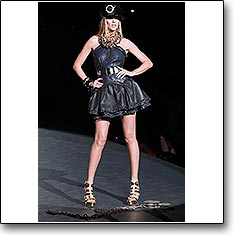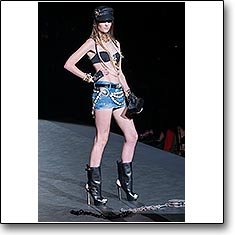D Squared 2 Spring Summer '13 Code: d-squared-s1310 model: Anne Vyalitsyna D Squared 2 Spring Summer '13 Code: d-squared-s1311 model: Melissa Tammerijn D Squared 2 Spring Summer '13 Code: d-squared-s1312 model: Montana Cox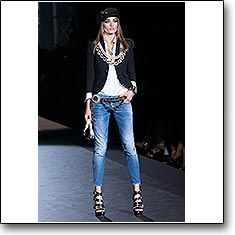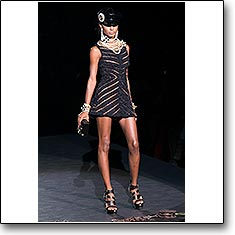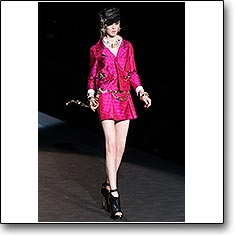D Squared 2 Spring Summer '13 Code: d-squared-s1313 model: Andreea Diaconu D Squared 2 Spring Summer '13 Code: d-squared-s1314 model: Jasmine Tookes D Squared 2 Spring Summer '13 Code: d-squared-s1315 model: Jemma Baines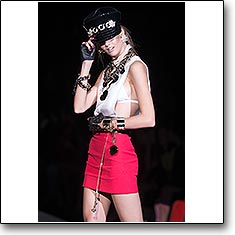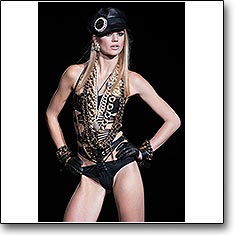D Squared 2 Spring Summer '13 Code: d-squared-s1316 model: Kendra Spears D Squared 2 Spring Summer '13 Code: d-squared-s1317 model: Toni Garrn D Squared 2 Spring Summer '13 Code: d-squared-s1318 model: Milou Sluis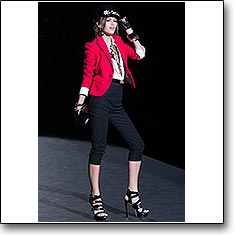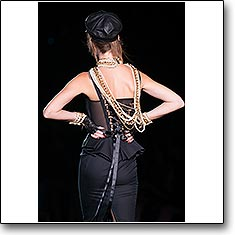D Squared 2 Spring Summer '13 Code: d-squared-s1319 D Squared 2 Spring Summer '13 Code: d-squared-s1320 D Squared 2 Spring Summer '13 Code: d-squared-s1321 model: Ava Smith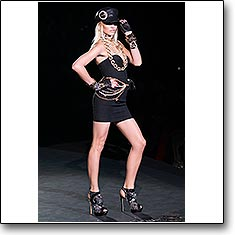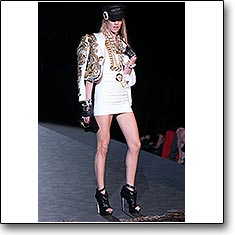D Squared 2 Spring Summer '13 Code: d-squared-s1322 model: Ginta Lapina D Squared 2 Spring Summer '13 Code: d-squared-s1323 model: Lindsey Wixson D Squared 2 Spring Summer '13 Code: d-squared-s1324 model: Julia Frauche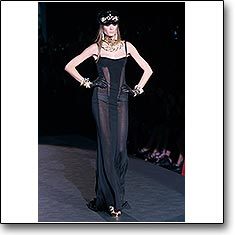D Squared 2 Spring Summer '13 Code: d-squared-s1325 model: Jessica Stam D Squared 2 Spring Summer '13 Code: d-squared-s1326 model: Jac Jagaciak D Squared 2 Spring Summer '13 Code: d-squared-s1327 model: Irina Kulikova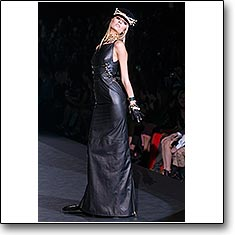D Squared 2 Spring Summer '13 Code: d-squared-s1328 model: Cara Delevingne D Squared 2 Spring Summer '13 Code: d-squared-s139 model: Bette Franke D Squared 2 Spring Summer '13 Code: d-squared-s1330 model: Constance JablonskiD Squared 2 Spring Summer '13 Code: d-squared-s1331 D Squared 2 Spring Summer '13 Code: d-squared-s1332 D Squared 2 Spring Summer '13 Code: d-squared-s1333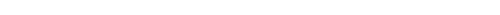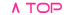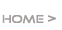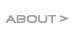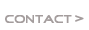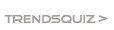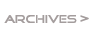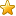Favourites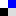Del.icio.us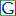Google BookmarksFacebook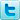Twitter